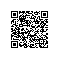# thinkphp对数据库操作有哪些内置函数

getModelName() 获取当前Model的名称
getTableName() 获取当前Model的数据表名称
switchModel(type,vars=array()) 动态切换模型
table() 设置当前操作的数据表
field() 设置要查询的数据字段
where() 设置查询或者操作条件
data(data) 设置数据对象
order(order) 设置排序
limit(limit) 查询限制
page(page) 查询分页
join(join) 进行JOIN查询
having(having) 进行having查询
group(group) 进行group查询
lock(lock) 查询锁定
distinct(distinct) 唯一性查询
count(field) 记录统计
sum(field) 总数查询
min(field) 最小值查询
max(field) 最大值查询

avg(field) 平均值查询
_initialize() 模型初始化方法
_before_write(&data) 写入数据前的回调方法 包括新增和更新
_before_insert(&data,options) 写入数据前的回调方法
_after_insert(data,options) 写入数据后的回调方法
save(data='',options=array()) 更新数据到数据库
_before_update(&data,options) 更新数据前的回调方法
_after_update(data,options) 更新成功后的回调方法
delete(options=array()) 删除数据
_after_delete(data,options) 删除成功后的回调方法
select(options=array()) 查询数据集
_after_select(&resultSet,options) 查询成功后的回调方法
findAll(options=array()) select方法的别名
_options_filter(&options) 表达式过滤回调方法
find(options=array()) 查询数据
_after_find(&result,options) 查询成功的回调方法
setField(field,value,condition='') 设置记录的某个字段值
setInc(field,condition='',step=1) 字段值增长
setDec(field,condition='',step=1) 字段值减少
getField(field,condition='',sepa=' ') 获取某个字段值
create(data='',type='') 创建数据对象

autoCheckToken(data) 表单令牌验证

query(sql) 执行原生SQL查询

execute(sql='') 执行原生SQL操作

startTrans() 启动事务

commit() 提交事务

rollback() 事务回滚

getError() 获取模型的错误信息

getDbError() 获取数据库的错误信息

getLastInsID() 获取最后执行的SQL语句

getPk() 获取主键名称

getDbFields() 获取数据表的字段信息

regex(value,rule) 使用正则验证数据

setProperty(name,value) 设置模型的属性值

2.1版新增方法:

topN(count,options=array()) 查询满足条件的前N个记录

getN(position=0,options=array()) 查询符合条件的第N条记录

0 表示第一条记录 -1 表示最后一条记录

first(options=array()) 获取满足条件的第一条记录

last(options=array()) 获取满足条件的最后一条记录

returnResult(data,type='') 返回指定的数据类型

setLazyInc(field,condition='',step=1,lazyTime=0) 字段值延迟增长

setLazyDec(field,condition='',step=1,lazyTime=0) 字段值延迟减少

patchQuery(sql=array()) 批处理执行SQL语句

getPartitionTableName(data=array()) 得到分表的的数据表名使用钉钉扫一扫加入圈子
+ 订阅